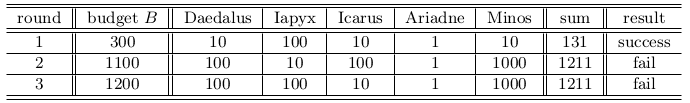D - D as in Daedalus

Languages: C, C++, Java, Python, ... (details)

Daedalus is playing the game of “Don’t be greedy”, in which $N$ players sit around a table having each of them five cards labelled $1$, $10$, $100$, $1000$ and $10000$ points. In “Don’t be greedy” the players may not talk to each other once the game starts, and there are $M$ rounds. In each round, the bank announces a budget $B$. Then each player chooses one of the cards and places it, face down, on the table. The bank then turns the cards, so that all players can see all $N$ cards. If the sum of the points in the chosen cards is less than or equal to $B$, then the bank gives to each player exactly the amount of points in the card he or she chose. Otherwise, no one gets anything. Each player gets his or her card back before the next round. The players are very rational and would like to maximize their points and minimize their regrets! What would you do in this situation? Cooperate or defect?

Take the following table as an example. Daedalus won a total of $10$ points, in the end, because only the first round was successful. But, looking back on the game, he sees that he could have won $110$ points, if he had chosen $100$ points in the first round and $10$ points in the third round. That is, Daedalus could have won $100$ extra points! This holds only, of course, assuming the cards chosen by the other players remain unchanged.Given the budget and the cards chosen in each round, we need to compute the maximum total number of extra points Daedalus could have won, in the end, if he had chosen the best possible card in each round, assuming the cards chosen by the other players remain unchanged.

Input

The first line contains two integers $N$ and $M$ , representing respectively the number of players and the number of rounds ($1 \leq N \leq 20$ and $1 \leq M \leq 50$). Each of the next $M$ lines describes a round with an integer $B$ indicating the budget ($1 \leq B \leq 10^6$), followed by $N$ integers $C_1, C_2, . . . , C_N$ representing that the $i$-th player chose the card labelled with $C_i$ points during that round $(C_i \in \{1, 10, 100, 1000, 10000\}$ for $i = 1, 2, ..., N)$. Daedalus is the first player.

Output

Output a line with an integer representing the maximum total number of extra points Daedalus could have won, if he had chosen the best possible card in each round, assuming the cards chosen by the other players remain unchanged.

Sample test(s)

Input
5 3 300 10 100 10 1 10 1100 100 10 100 1 1000 1200 100 100 10 1 1000
Output
100
Input
3 2 2000 1000 1000 1000 21 1 1 10
Output
9